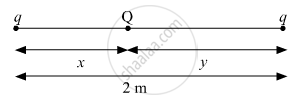# Answer the following question. Two identical point charges, q each, are kept 2m apart in the air. A third point charge Q of unknown magnitude and sign is placed on the line joining the charges - Physics

Numerical

Two identical point charges, q each, are kept 2m apart in the air. A third point charge Q of unknown magnitude and sign is placed on the line joining the charges such that the system remains in equilibrium. Find the position and nature of Q.

#### Solution(K(q)(Q))/x = (-K(q)(q))/2

⇒ Q = (-qx)/2

because (KqQ)/x = (KQq)/y

x = y

x + y = 2

∴ x = y = 1

Q = (-q)/2

Concept: Equipotential Surfaces
Is there an error in this question or solution?